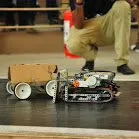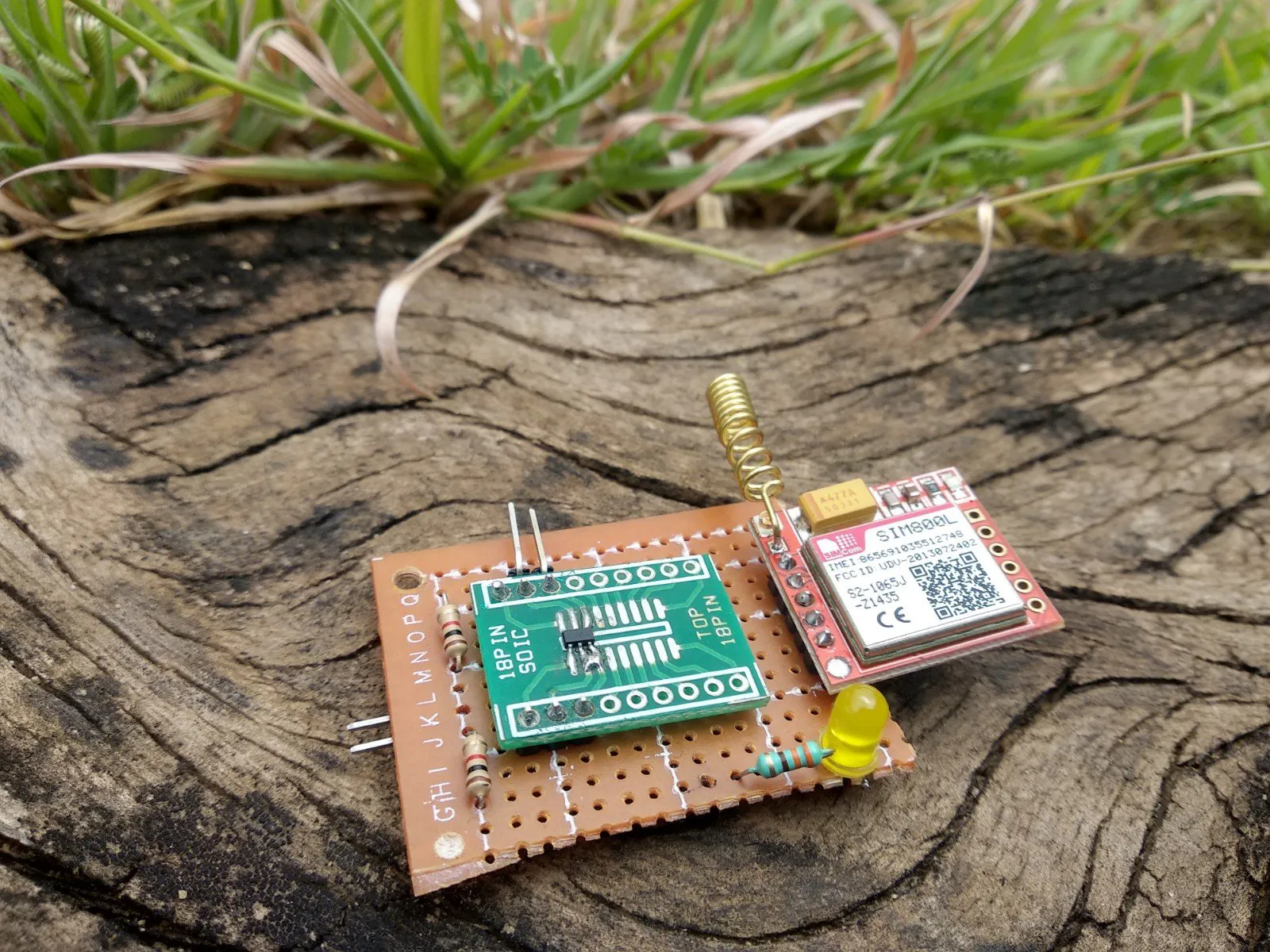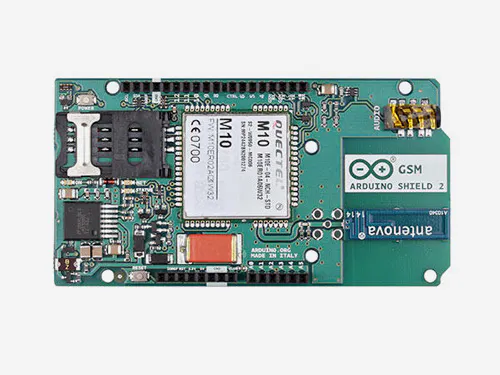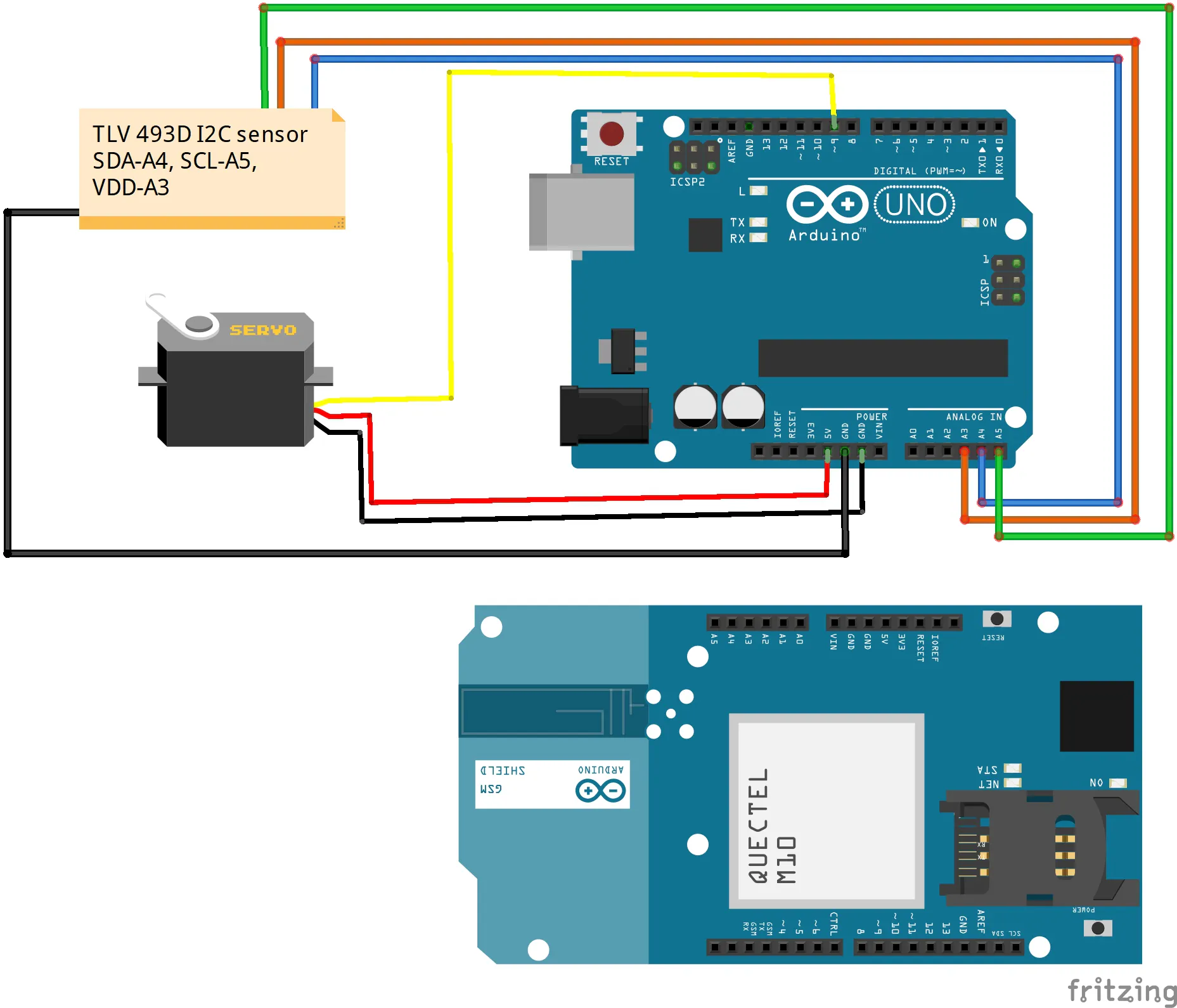# IEncode - Intelligent Encoder for Industrial Motors

Predictive maintenance of industrial motors using high precision 3D magnetic sensor TLV493D-A1B6.

BeginnerFull instructions provided1 hour1,618## Things used in this project

### Hardware componentsArduino UNO & Genuino UNO
×1Arduino GSM shield V2
×1
 Infineon TLV 493D A1B6
×1

### Software apps and online servicesArduino IDE

## Custom parts and enclosures

### Log data

Log data in xlsx format

## Schematics

### Schematic

Schematic Diagram## Code

### IEncode

C/C++
```#include <SoftwareSerial.h>
#include "TLV493D.h"
#include "Wire.h"

TLV493D sensor2;
int i = 0;
int threshold = 50;
int val = 0;
int xyang = 0;
int yzang = 0;
int zxang = 0;
float v1, v2 = 0;
const int sensor2_pwr_pin = A3;
const int i2c_sda = A4;
SoftwareSerial sim(10, 11);
int _timeout;
String _buffer;
String number = "+919482005201";
void setup() {
pinMode(sensor2_pwr_pin, OUTPUT);
pinMode(i2c_sda, OUTPUT);
pinMode(5, OUTPUT);
digitalWrite(sensor2_pwr_pin, LOW);
digitalWrite(i2c_sda, LOW);
//init sensor2
digitalWrite(sensor2_pwr_pin, HIGH);
digitalWrite(i2c_sda, HIGH); //0x5E
Serial.println("Starting sensor 2");
delay(500);

Wire.begin(); // Begin I2C wire communication

//initialize sensor 2
//  Serial.print("Initializing sensor 2: 0x");
Serial.println(sensor2.init(HIGH), HEX);
delay(7000); //delay for 7 seconds to make sure the modules get the signal
Serial.begin(9600);
_buffer.reserve(50);
// Serial.println("Sistem Started...");
sim.begin(9600);
delay(1000);
}
void loop()
{
xyang = ( sensor2.m_dPhi_xy * 57.2958); // Measures angle between X and Y i.e encoder angle or shaft angle
Serial.print("X angle == ");
Serial.print(xyang);
v1 = sensor2.m_dBz;
if (v1 > threshold)
{
i++;
}
if (i > 5)
{
digitalWrite(5,HIGH);
SendMessage();// Sends an SMS to the concerned person or a soft number that machine needs a service
i = 0;
}

}
void SendMessage()
{
//Serial.println ("Sending Message");
sim.println("AT+CMGF=1");    //Sets the GSM Module in Text Mode
delay(1000);
//Serial.println ("Set SMS Number");
sim.println("AT+CMGS=\"" + number + "\"\r"); //Mobile phone number to send message
delay(1000);
String SMS = "Service is required for machine number 5";
sim.println(SMS);
delay(100);
sim.println((char)26);// ASCII code of CTRL+Z
delay(1000);
}
_timeout = 0;
while  (!sim.available() && _timeout < 12000  )
{
delay(13);
_timeout++;
}
if (sim.available()) {
}
}
```

### Servo Encoder

C/C++
Testing Encoder with Servo
```#include "TLV493D.h"
#include "Wire.h"
#include <Servo.h>
TLV493D sensor1;
TLV493D sensor2;

int val =0;
int xyang =0;
int yzang =0;
int zxang =0;
float v1,v2=0;
const int sensor2_pwr_pin = A3;
const int i2c_sda = A4;

Servo myservo;  // create servo object to control a servo
// twelve servo objects can be created on most boards

int pos = 0;

void setup()
{
Serial.begin(115200);

pinMode(sensor2_pwr_pin, OUTPUT);
pinMode(i2c_sda, OUTPUT);

digitalWrite(sensor2_pwr_pin, LOW);
digitalWrite(i2c_sda, LOW);

delay(500);

digitalWrite(sensor2_pwr_pin, HIGH);
digitalWrite(i2c_sda, HIGH); //0x5E
// Serial.println("Starting sensor 2");
delay(500);

Wire.begin(); // Begin I2C wire communication
myservo.attach(9); // Servo initilisation

//initialize sensor 2
//  Serial.print("Initializing sensor 2: 0x");
Serial.println(sensor2.init(HIGH), HEX);
}

void loop()
{
for (pos = 0; pos <= 180; pos += 1) { // goes from 0 degrees to 180 degrees
// in steps of 1 degree
//Serial.print("Servo angle=");// Servo angle printing for comparision
// Serial.println(pos);
myservo.write(pos);              // tell servo to go to position in variable 'pos'
delay(15);                       // waits 15ms for the servo to reach the position
sensor2.update();

// Serial.print(sensor2.m_dBz);

xyang=( sensor2.m_dPhi_xy*57.2958);//Converts radian value to degree
// Serial.print("X angle == ");
Serial.println(xyang);// Calculated angle between X and Y plane
// Serial.println(";");//\t");
/*yzang=( sensor2.m_dPhi_yz*57.2958);//
Serial.print("Y angle ==");
Serial.print(yzang);
Serial.println(";");//\t");
zxang=( sensor2.m_dPhi_xz*57.2958);
Serial.print("Z angle ==");
Serial.print(zxang);
Serial.println(";");//\t");
//  Serial.println(";");
// Serial.println(sensor2.m_dMag_2);
delay(20);*/
delay(200);
}
for (pos = 180; pos >= 0; pos -= 1) { // goes from 180 degrees to 0 degrees
myservo.write(pos);
//      Serial.print("Servo angle=");
// Serial.println(pos);
delay(15);
sensor2.update();

//  Serial.print(sensor2.m_dBz);

xyang=( sensor2.m_dPhi_xy*57.2958);
//  Serial.print("X angle == ");
Serial.println(xyang);
//  Serial.println(";");//\t");
/* yzang=( sensor2.m_dPhi_yz*57.2958);
Serial.print("Y angle ==");
Serial.print(yzang);
Serial.println(";");//\t");
zxang=( sensor2.m_dPhi_xz*57.2958);
Serial.print("Z angle ==");
Serial.print(zxang);
Serial.println(";");//\t");
//  Serial.println(";");
// Serial.println(sensor2.m_dMag_2);
delay(500);
// waits 15ms for the servo to reach the position*/
delay(200);
}
}

```